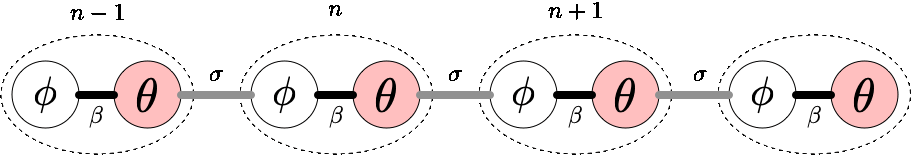EXPLORABLES

This explorable illustrates the wealth of dynamical states exhibited in a network of Janus Oscillators. Janus oscillators are a generalization of ordinary phase coupled oscillators (see Complexity Explorable "Ride my Kuramotocycle!"). Like the Roman god Janus, a Janus oscillator has two "faces", each corresponding to a distinct internal phase variable. This slight generalization produces a surprisingly large array of complex phenomena in a simple ring arrangement.

Press play and keep on reading...

## This is how it works

The building blocks of this system are Janus Oscillators. Their state is defined by two phase variables $$\theta(t)$$ and $$\phi(t)$$. Without any (internal or external) forces, these phases advance at constant angular speeds: $$\dot\theta = \omega$$ and $$\dot\phi= \nu$$. One natural frequency is larger than the other $$\omega>\nu$$, like a clock with hands moving at different speeds. If you want to estabilish an intuitive mental image of the system: i) click on the clock view, ii) select e.g. 8 oscillators, iii) turn off the interaction, and iv) press play.

The system contains $$N$$ such oscillators (labeled by $$n$$) arranged on a ring. The dynamics of the system is defined by the rate of change of the $$2N$$ angle variables $$\theta _n(t)$$ and $$\phi _n(t)$$:

$\dot\theta_{n} = \omega_{n}+\beta \sin(\phi_{n}-\theta_{n})+\sigma \sin(\phi_{n+1}-\theta_{n})$

$\dot\phi_{n} = \nu_{n}+\beta \sin(\theta_{n}-\phi_{n})+\sigma \sin(\theta_{n-1}-\phi_{n}).$

The parameter $$\beta$$ quantifies the magnitude of the internal coupling. For sufficiently large $$\beta$$, an oscillator will phase lock internally: both phases advancing at identical angular velocity with a constant phase difference.

With parameter $$\sigma$$ you can tune the interaction between neighboring oscillators along the ring in such a way that the fast phase of one oscillator tries to phase align with the slow phase of one of the neighboring oscillators.By default all oscillators have identical frequency pairs $$(\omega _n,\nu _n)=(\omega,\nu)$$. By increasing the heterogeneity the variation of natural frequency pairs between oscillators increases.

### The display: "What are those moving dots?"

Each oscillator is depicted as a moving dot at a location given by the complex number

$z_n(t)=\frac{1}{2}\left(e^{i\theta_{n}(t)}+e^{i\phi_{n}(t)}\right)$

considering the display as the complex plane. When the alignment of internal phases is perfect $$|z_n(t)|$$ has unit length and will just be rotating at the mean angular velocity of the oscillator. If the internal phases differ by $$180\degree$$ then $$z(t)=0$$. For phase locked oscillators the length $$|z_n(t)|$$ remains constant. The color quantifies the total speed at which an oscillator changes its state, i.e. the quantity $$|\dot\theta_{n}(t)+\dot\phi_{n}(t)|$$ (red=fast, black=slow). The symbol size quantifies the speed of the difference in internal phases, i.e. the quantity $$|\dot\theta_{n}(t)-\dot\phi_{n}(t)|$$.

Depending on the parameter choice, it may take a while for the system to equilibrate. If you are impatient, you can press the fast-forward button to advance time by larger chunk of timesteps.

If you prefer, you can select the clock view in which each oscillators' phases are depicted as hand on a clock. This is a bit more intuitive and less abstract but structures in the dynamics can't be seen as easily.

Some dynamic states are more salient when you go into the moving reference frame in which the entire plane is rotated at the average angular velocity of the oscillators.

## Try this

Many different behaviors can be observed for different parameter regimes. Follow the guides below to explore some of them:

### Chimera states

Press the reset parameters button to set the coupling constants to intermediate values and the heterogeneity to zero. The asynchronous state appears initially as dots move in a disordered fashion, passing near the origin and near the exterior occasionally. This state is transient, it takes a while for the system to converge. Press the fast-forward button several times and see if the system settles into an ordered or disordered state.

You are likely to see a chimera state, in which a large group of oscillators phase lock and move in unison around a circle, while a smaller number of oscillators continue to move in a disordered fashion. You can select the chimera preset to generate one of them.

### Explosive synchronization

Select the asynchronous preset. The system will remain in a disordered state. This asynchronous state vanishes abruptly if the coupling constant $$\sigma$$ increases slightly. This transition is analogous to a first-order phase transition, and the system exhibits hysteresis. Again, press the fast-forward if you want to see the final state.

### Marginally stable remote synchronization

Select the phase-locked preset. Start decreasing the coupling constant $$\sigma$$. The synchronized state will persist at smaller radii until the oscillators start to bounce! Switch to the clock view to see what's going on. All the red hands are synchronized, and all the gray hands are synchronized, even though the red angles are coupled only to gray ones, and vice versa. This is called a remotely synchronized state, since the synchronization is mediated though intermidiate connections. This state may appear to be long-lived, but it is not an attractor! If you click the fast-forward button enough times, disorder slowly emerges and the system decays to the asynchronous state.

### Asymmetry-induced synchronization

Next consider the surprising results of increasing the heterogeneity. First, select the chimera preset. Increase the heterogeneity a tiny amount and press the fast-forward button several times. The chimera state deforms slightly but remains essentially unchanged. Continue moving the heterogeneity up and pressing fast-forward repeatedly. Eventually, you will see that the chimera falls apart and the system may phase-lock. Surprisingly, increasing the heterogeneity in the ring increases the propensity of the oscillators to synchronize.

### Chimera intermittency

Select the intermittency preset to set the heterogeneity to a large value and the coupling constant $$\sigma$$ to an intermediate value. States that resemble chimeras begin to emerge, but the system will not settle down, as the chimeras keep falling apart! This state of chimera intermittency is stabilized only when the heterogeneity is sufficiently large.

## Further reading

Z. G. Nicolaou, D. Eroglu, and A. E. Motter. Multifaceted dynamics of Janus oscillator networks. Phys. Rev. X 9 (2019).